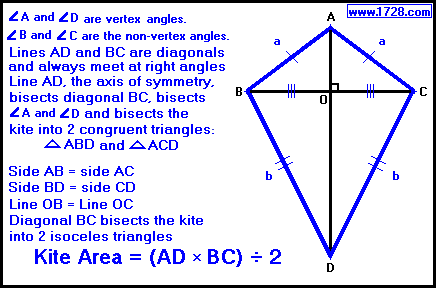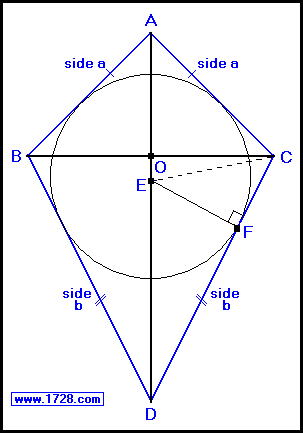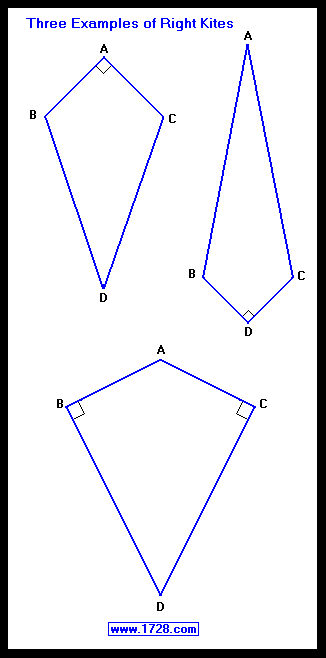Kite Calculator
Scroll Down for instructions and definitionsAll kites are quadrilaterals with the following properties: • no concave (greater than 180°) internal angles • no parallel sides • two pairs of equal, adjacent sides (a and b) • two equal angles (B and C) called non-vertex angles • diagonals which always meet at right angles • a diagonal, called the axis of symmetry (line AD), that bisects the other diagonal (line BC), bisects the vertex angles (A and D) and divides the kite into two congruent triangles (ABD and ACD) • a diagonal (line BC) that divides the kite into two isoceles triangles (ABC and BCD)
 If you know 3 data items of a kite, click on one of the eight buttons above that correspond to the 3 data items you know. Enter those numbers and then click "CALCULATE" to see the answers.The last two output boxes ("Line AE" and "radius") are for inscribing a circle within a kite. Read the next paragraph for more information.

 Inscribing A Circle Within A Kite All kites are tangential quadrilaterals, meaning that they are 4 sided figures into which a circle (called an incircle) can be inscribed such that each of the four sides will touch the circle at only one point. (Basically, this means that the circle is tangent to each of the four sides of the kite.) To inscribe a circle graphically (using compass and straight edge) within a kite: • draw the axis of symmetry AD • bisect one of the non-vertex angles (B or C) and extend this line so that it meets line AD at point E • from point E, draw a perpendicular to any of the four sides. • Inscribe the circle using point E as its center and line EF as its radius. Describing this mathematically: • Angle ACE = Angle ECD = ½ Angle ACD • Angle ACO = 90º - ½ Angle BAC • Angle OCE = Angle ACE - ACO • OE = ½ Line BC x tan (OCE) • AE = OA + OE • ED = AD -AE • Angle EDF = ½ Angle BDC • EF = sin (EDF) x ED

There is one special type of kite called the "right kite" which contains one or two right angles.
The image below shows all cases where the right angle may appear.Significant Figures >>> The default setting is for 5 significant figures but you can change that by inputting another number in the box above. Answers are displayed in scientific notation and for easier readability, numbers between .001 and 1,000 will be displayed in standard format (with the same number of significant figures.) The answers should display properly but there are a few browsers that will show no output whatsoever. If so, enter a zero in the box above. This eliminates all formatting but it is better than seeing no output at all.